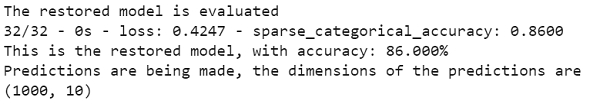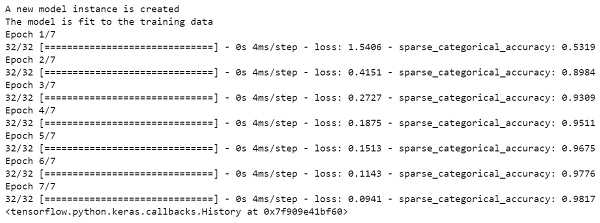# How can Keras be used to evaluate the restored model using Python?

PythonServer Side ProgrammingProgramming

Tensorflow is a machine learning framework that is provided by Google. It is an open−source framework used in conjunction with Python to implement algorithms, deep learning applications and much more. It is used in research and for production purposes. It has optimization techniques that help in performing complicated mathematical operations quickly.

The ‘tensorflow’ package can be installed on Windows using the below line of code −

pip install tensorflow

Keras was developed as a part of research for the project ONEIROS (Open ended Neuro−Electronic Intelligent Robot Operating System). Keras is a deep learning API, which is written in Python. It is a high−level API that has a productive interface that helps solve machine learning problems.

It runs on top of Tensorflow framework. It was built to help experiment in a quick manner. It provides essential abstractions and building blocks that are essential in developing and encapsulating machine learning solutions. It is highly scalable, and comes with cross platform abilities. This means Keras can be run on TPU or clusters of GPUs. Keras models can also be exported to run in a web browser or a mobile phone as well.

Keras is already present within the Tensorflow package. It can be accessed using the below line of code.

import tensorflow
from tensorflow import keras

We are using the Google Colaboratory to run the below code. Google Colab or Colaboratory helps run Python code over the browser and requires zero configuration and free access to GPUs (Graphical Processing Units). Colaboratory has been built on top of Jupyter Notebook. Following is the code −

## Example

print("The restored model is evaluated")
loss, acc = new_model.evaluate(test_images, test_labels, verbose=2)
print('This is the restored model, with accuracy: {:5.3f}%'.format(100 * acc))
print("Predictions are being made, the dimensions of the predictions are")
print(new_model.predict(test_images).shape)
print("A new model instance is created")

model = create_model()

print("The model is fit to the training data")
model.fit(train_images, train_labels, epochs=7)

## Output## Explanation

• The restored model is evaluated using the ‘evaluate’ method.

• Its accuracy and loss during training is determined.

• These values are displayed on the console.

• The predictions are made using the ‘predict’ method.

• The dimensions of the test data are displayed on the console.

• Another new instance of the model is created using the ‘create_model’ method.

• The model is fit to the training data in 7 epochs.